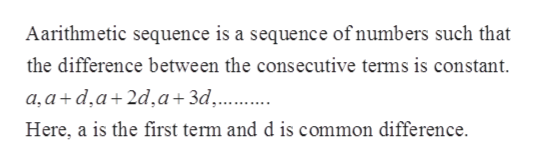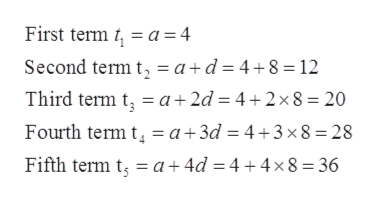# Determine the first five terms of the arithmetic sequence {an} with a1 = 4, and d = 8.

Question
2 views

Determine the first five terms of the arithmetic sequence {an} with a1 = 4, and d = 8.

check_circle

Step 1

Given: to find first five terms of the arithmetic sequence with a1 = 4, and d = 8.

Step 2

Concept used:help_outlineImage TranscriptioncloseAarithmetic sequence is a sequence of numbers such that the difference between the consecutive terms is constant. a,a+d,a+2d,a+3d¸..... Here, a is the first term and d is common difference. fullscreen
Step 3

Given the first term a=4 and common difference is d = 8.

...help_outlineImage TranscriptioncloseFirst term t, = a = 4 Second term t, = a+d = 4+8 = 12 Third term t, = a+ 2d = 4+2× 8 = 20 = a+3d = 4+3×8=28 Fourth term t Fifth term t, = a+4d = 4 +4×8=36 fullscreen

### Want to see the full answer?

See Solution

#### Want to see this answer and more?

Solutions are written by subject experts who are available 24/7. Questions are typically answered within 1 hour.*

See Solution
*Response times may vary by subject and question.
Tagged in

### Sequence and Series